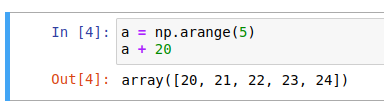# Incorrect Answer in Data Analysis with Python

In the Numpy Operations page.

I’ve read several existing posts here that justify the accepted answer is [0, 1, 2, 3, 4] because the “a + 20” is not being assigned and then printed.

However, the topic is taught using Jupyter Labs, all the examples are in it, and putting in the exact code as it appears in the question gives you:Regardless, this question could use clarification. Either specify that this question is in colabs/juypter or in normal python.

IMO if it really is a ‘trick’ question (i.e not about the arithmetic and actually about syntax), it’s not quite appropriate this early, as we are still in an introduction to numpy.

This has nothing to do with numpy. This is a question of if you understand introductory Python syntax. Understanding the difference between assignment and simply printing output is critical in notebooks. The correct answer is that `a` is set on the first line and remains unchanged after the second line is executed.

What is the value of `a` after you run the following code?

The value is determined by the first line of the provided code.

This topic was automatically closed 182 days after the last reply. New replies are no longer allowed.